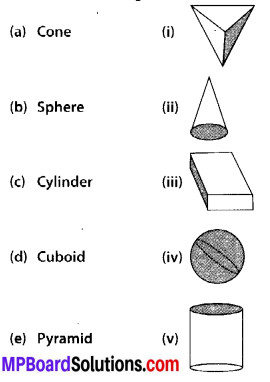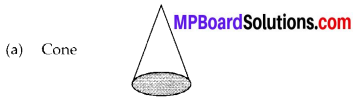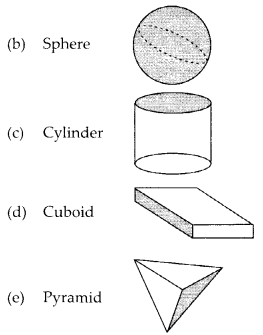# MP Board Class 6th Maths Solutions Chapter 5 Understanding Elementary Shapes Ex 5.9

## MP Board Class 6th Maths Solutions Chapter 5 Understanding Elementary Shapes Ex 5.9

Question 1.
Match the following :Give two new examples of each shape.
Solution:
(a) ➝ (ii)
(b) ➝ (iv)
(c) ➝ (v)
(d) ➝ (iii)
(e) ➝ (i)Two examples of cone : Ice-cream, Birthday cap
Two examples of sphere : Ball, Rasgulla
Two example of Cylinder : Pipe, Can
Two examples of Cuboid : Box, Brick
Two examples of a Pyramid : Roof of the house, Cheese graterQuestion 2.
What shape is
(a) Your instrument box?
(b) A brick?
(c) A match box?